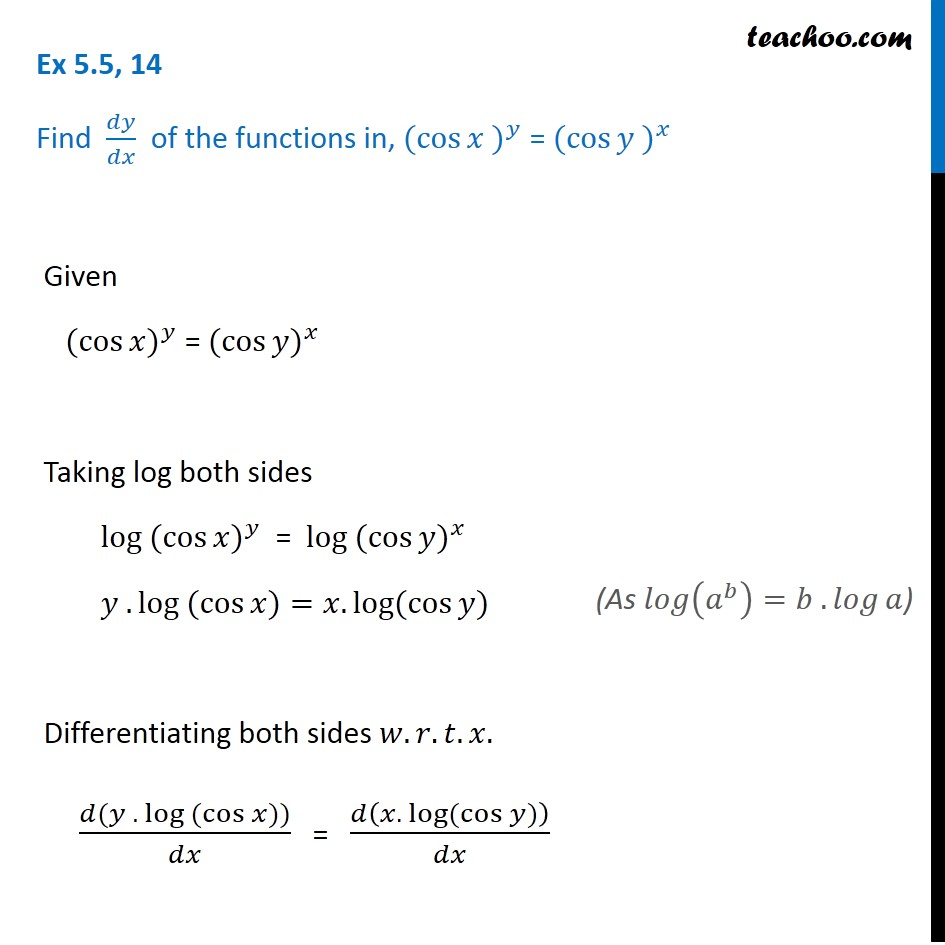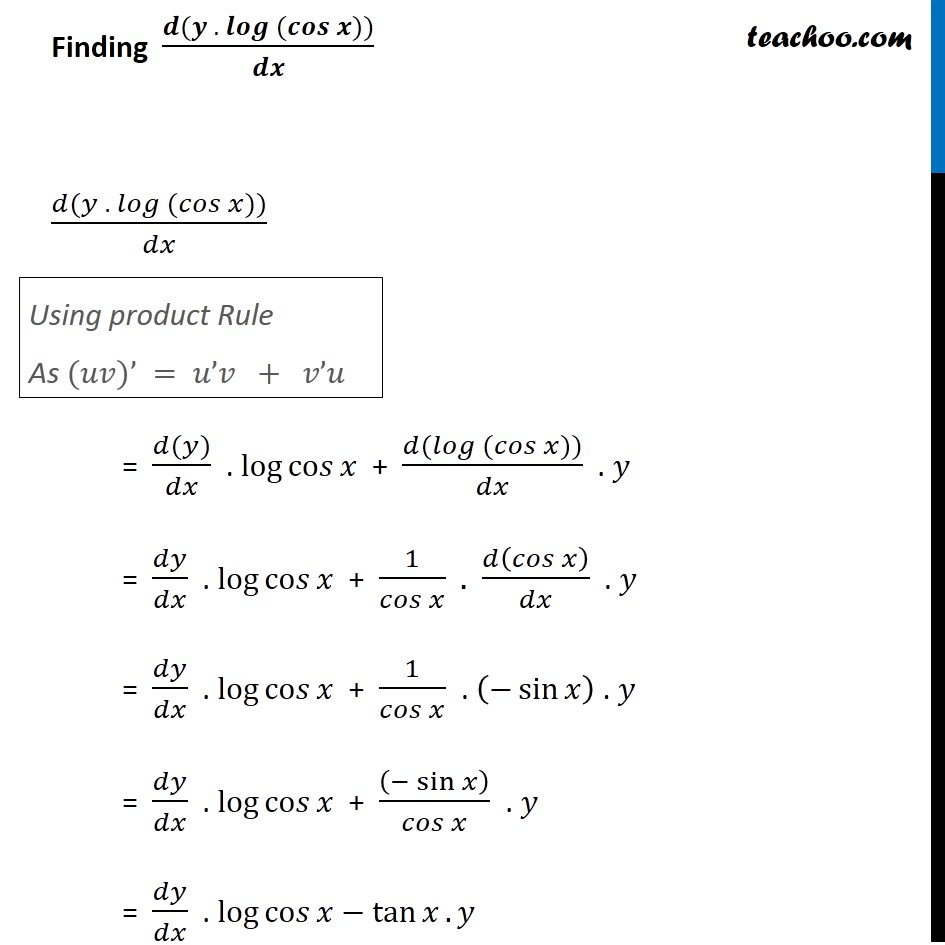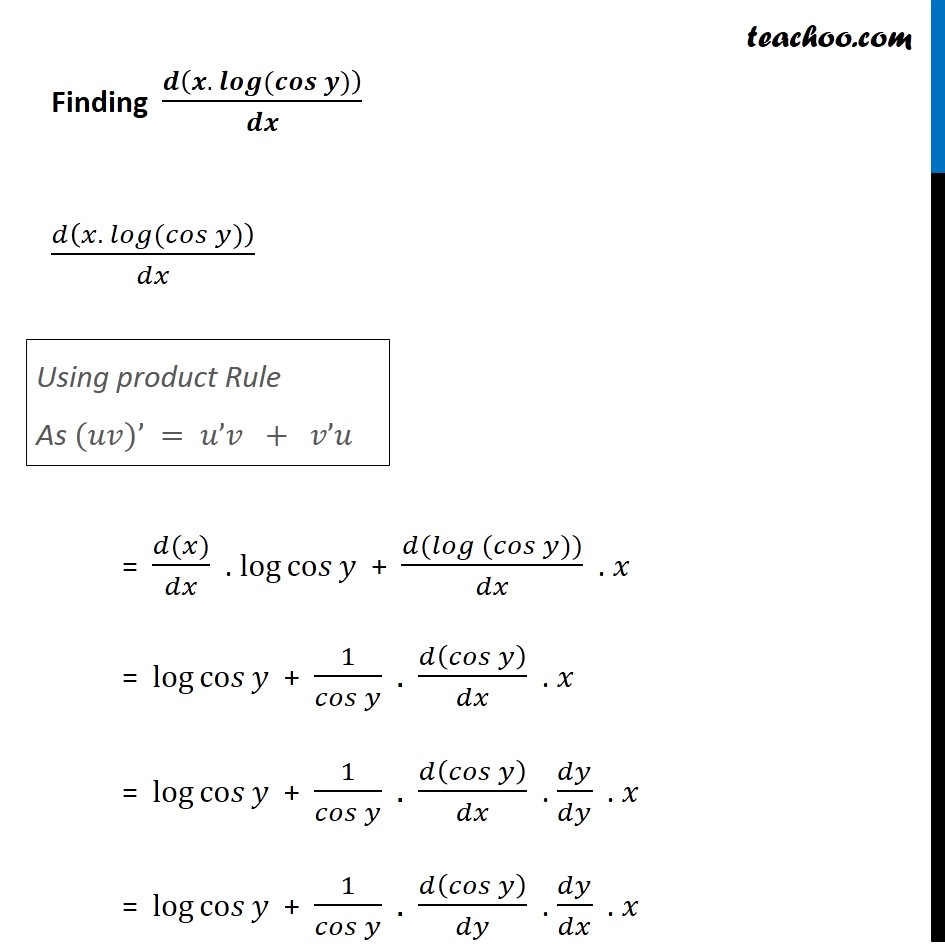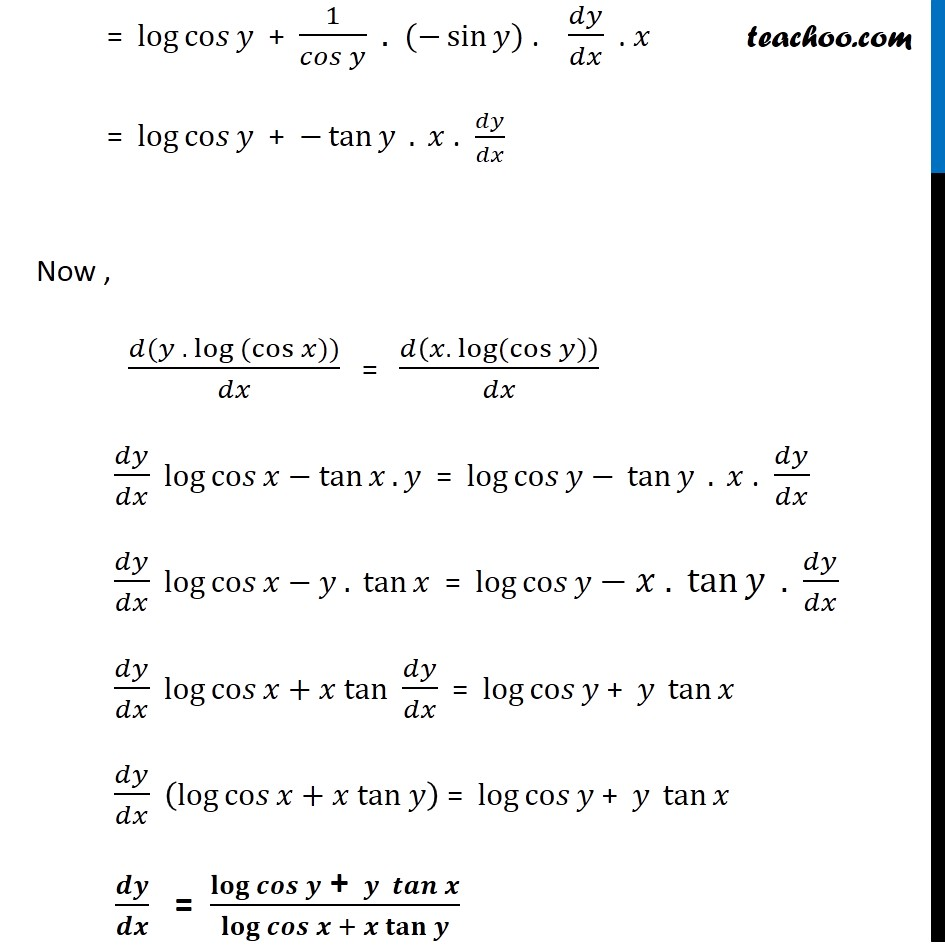Check Full Chapter Explained - Continuity and Differentiability - https://you.tube/Chapter-5-Class-12-Continuity1. Chapter 5 Class 12 Continuity and Differentiability
2. Serial order wise
3. Ex 5.5

Transcript

Ex 5.5, 14 Find 𝑑𝑦/𝑑𝑥 of the functions in, 〖(cos⁡〖𝑥 〗)〗^𝑦 = 〖(cos⁡〖𝑦 〗)〗^𝑥 Given 〖(cos⁡𝑥)〗^𝑦 = 〖(cos⁡𝑦)〗^𝑥 Taking log both sides log 〖(cos⁡𝑥)〗^𝑦 = log 〖(cos⁡𝑦)〗^𝑥 𝑦 . log (cos⁡𝑥)=𝑥.log⁡〖(cos⁡𝑦)〗 Differentiating both sides 𝑤.𝑟.𝑡.𝑥. (𝑑(𝑦 . log (cos⁡𝑥)))/𝑑𝑥 = 𝑑(𝑥.〖 log〗⁡〖(cos⁡𝑦)〗 )/𝑑𝑥 (As 𝑙𝑜𝑔⁡(𝑎^𝑏 )=𝑏 . 𝑙𝑜𝑔⁡𝑎) Finding (𝒅(𝒚 . 𝒍𝒐𝒈 (𝒄𝒐𝒔⁡𝒙)))/𝒅𝒙 (𝑑(𝑦 . 𝑙𝑜𝑔 (𝑐𝑜𝑠⁡𝑥)))/𝑑𝑥 = (𝑑(𝑦))/𝑑𝑥 . log co𝑠⁡𝑥 + (𝑑(𝑙𝑜𝑔 (𝑐𝑜𝑠⁡𝑥)))/𝑑𝑥 . 𝑦 = 𝑑𝑦/𝑑𝑥 . log co𝑠⁡𝑥 + 1/𝑐𝑜𝑠⁡𝑥 . 𝑑(𝑐𝑜𝑠⁡𝑥 )/𝑑𝑥 . 𝑦 = 𝑑𝑦/𝑑𝑥 . log co𝑠⁡𝑥 + 1/𝑐𝑜𝑠⁡𝑥 . (−sin⁡𝑥 ) . 𝑦 = 𝑑𝑦/𝑑𝑥 . log co𝑠⁡𝑥 + ((−sin⁡𝑥 ))/𝑐𝑜𝑠⁡𝑥 . 𝑦 = 𝑑𝑦/𝑑𝑥 . log co𝑠⁡𝑥−tan⁡𝑥. 𝑦 Using product Rule As (𝑢𝑣)’ = 𝑢’𝑣 + 𝑣’𝑢 Finding 𝒅(𝒙.〖 𝒍𝒐𝒈〗⁡〖(𝒄𝒐𝒔⁡𝒚)〗 )/𝒅𝒙 𝑑(𝑥.〖 𝑙𝑜𝑔〗⁡〖(𝑐𝑜𝑠⁡𝑦)〗 )/𝑑𝑥 = (𝑑(𝑥))/𝑑𝑥 . log co𝑠⁡𝑦 + (𝑑(𝑙𝑜𝑔 (𝑐𝑜𝑠⁡𝑦)))/𝑑𝑥 . 𝑥 = log co𝑠⁡𝑦 + 1/𝑐𝑜𝑠⁡𝑦 . 𝑑(𝑐𝑜𝑠⁡𝑦 )/𝑑𝑥 . 𝑥 = log co𝑠⁡𝑦 + 1/𝑐𝑜𝑠⁡𝑦 . 𝑑(𝑐𝑜𝑠⁡𝑦 )/𝑑𝑥 . 𝑑𝑦/𝑑𝑦 . 𝑥 = log co𝑠⁡𝑦 + 1/𝑐𝑜𝑠⁡𝑦 . 𝑑(𝑐𝑜𝑠⁡𝑦 )/𝑑𝑦 . 𝑑𝑦/𝑑𝑥 . 𝑥 Using product Rule As (𝑢𝑣)’ = 𝑢’𝑣 + 𝑣’𝑢 = log co𝑠⁡𝑦 + 1/𝑐𝑜𝑠⁡𝑦 . (−sin⁡𝑦) . 𝑑𝑦/𝑑𝑥 . 𝑥 = log co𝑠⁡𝑦 + −tan⁡𝑦 . 𝑥 . 𝑑𝑦/𝑑𝑥 Now , (𝑑(𝑦 . log (cos⁡𝑥)))/𝑑𝑥 = 𝑑(𝑥.〖 log〗⁡〖(cos⁡𝑦)〗 )/𝑑𝑥 𝑑𝑦/𝑑𝑥 log co𝑠⁡𝑥−tan⁡𝑥. 𝑦 = log co𝑠⁡𝑦 − tan⁡𝑦 . 𝑥 . 𝑑𝑦/𝑑𝑥 𝑑𝑦/𝑑𝑥 log co𝑠⁡𝑥−𝑦 . tan⁡𝑥 = log co𝑠⁡𝑦 − 𝑥 . tan⁡𝑦 . 𝑑𝑦/𝑑𝑥 𝑑𝑦/𝑑𝑥 log co𝑠⁡𝑥+𝑥 tan 𝑑𝑦/𝑑𝑥 = log co𝑠⁡𝑦 + 𝑦 tan⁡𝑥 𝑑𝑦/𝑑𝑥 (log co𝑠⁡𝑥+𝑥 tan 𝑦) = log co𝑠⁡𝑦 + 𝑦 tan⁡𝑥 𝒅𝒚/𝒅𝒙 = (𝐥𝐨𝐠 𝒄𝒐𝒔⁡𝒚 " + " 𝒚 𝒕𝒂𝒏⁡𝒙)/(𝐥𝐨𝐠 𝒄𝒐𝒔⁡𝒙 + 𝒙 𝐭𝐚𝐧 𝒚)

Ex 5.5